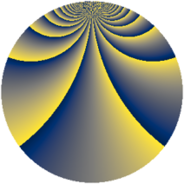# Properties

 Label 2736.2.gkLevel $2736$ Weight $2$ Character orbit 2736.gk Rep. character $\chi_{2736}(257,\cdot)$ Character field $\Q(\zeta_{18})$ Dimension $708$ Sturm bound $960$

# Related objects

## Defining parameters

 Level: $$N$$ $$=$$ $$2736 = 2^{4} \cdot 3^{2} \cdot 19$$ Weight: $$k$$ $$=$$ $$2$$ Character orbit: $$[\chi]$$ $$=$$ 2736.gk (of order $$18$$ and degree $$6$$) Character conductor: $$\operatorname{cond}(\chi)$$ $$=$$ $$171$$ Character field: $$\Q(\zeta_{18})$$ Sturm bound: $$960$$

## Dimensions

The following table gives the dimensions of various subspaces of $$M_{2}(2736, [\chi])$$.

Total New Old
Modular forms 2952 732 2220
Cusp forms 2808 708 2100
Eisenstein series 144 24 120

## Trace form

 $$708q + 6q^{3} - 9q^{5} + 6q^{7} - 12q^{9} + O(q^{10})$$ $$708q + 6q^{3} - 9q^{5} + 6q^{7} - 12q^{9} + 9q^{11} - 3q^{13} + 6q^{15} + 12q^{19} - 24q^{21} + 9q^{23} - 3q^{25} + 9q^{27} - 9q^{29} + 9q^{31} - 9q^{33} + 45q^{35} + 12q^{39} - 27q^{41} - 33q^{43} - 3q^{45} + 9q^{47} + 630q^{49} + 6q^{51} - 3q^{55} - 6q^{57} + 9q^{59} - 3q^{61} + 156q^{63} - 9q^{65} + 3q^{67} - 9q^{69} + 24q^{73} - 18q^{77} + 3q^{79} - 3q^{85} + 3q^{87} + 69q^{91} - 15q^{93} + 9q^{95} - 21q^{97} + 33q^{99} + O(q^{100})$$

## Decomposition of $$S_{2}^{\mathrm{new}}(2736, [\chi])$$ into newform subspaces

The newforms in this space have not yet been added to the LMFDB.

## Decomposition of $$S_{2}^{\mathrm{old}}(2736, [\chi])$$ into lower level spaces

$$S_{2}^{\mathrm{old}}(2736, [\chi]) \cong$$ $$S_{2}^{\mathrm{new}}(171, [\chi])$$$$^{\oplus 5}$$$$\oplus$$$$S_{2}^{\mathrm{new}}(342, [\chi])$$$$^{\oplus 4}$$$$\oplus$$$$S_{2}^{\mathrm{new}}(684, [\chi])$$$$^{\oplus 3}$$$$\oplus$$$$S_{2}^{\mathrm{new}}(1368, [\chi])$$$$^{\oplus 2}$$